With real-time center frequency and bandwidth control!

In one of the previous articles, we discussed how to implement a simple lowpass and a highpass filter using the first-order allpass filter. That filter had a real-time cutoff frequency control.

Now, we can take it to the next level and design a bandpass and a bandstop filter with a second-order allpass filter. This design will allow us to control the center frequency and the bandwidth (or alternatively, the Q factor) in real time!

We’ll discuss this design and its properties, listen to a few examples, and look at a sample Python implementation at the end.

1. What Is a Bandstop (Notch) Filter?
2. What Is a Bandpass Filter?
3. Recap: The Second-Order Allpass Filter
4. Allpass-Based Bandstop Filter
5. Allpass-Based Bandpass Filter
6. Applications
7. Summary

## What Is a Bandstop (Notch) Filter?

A bandstop filter (also called a notch filter) is a filter that attenuates frequencies in a certain frequency range.

This frequency range is determined by a center frequency and a bandwidth.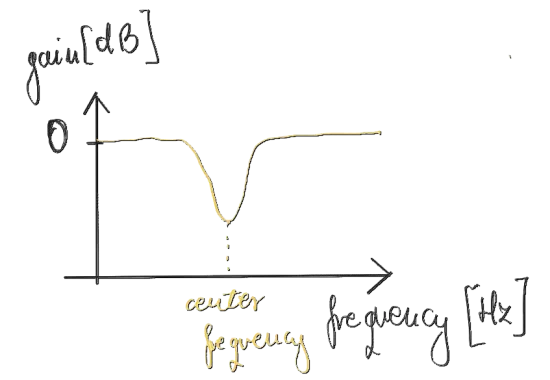Figure 1. Bandstop filter amplitude response.

The center frequency points to the frequency with the largest attenuation, the “dip” in the magnitude response of the filter.

The bandwidth determines how wide will the “dip” or “valley” be around the center frequency.

In order for the bandstop filter to sound equally wide in bandwidth at all center frequencies, we often use the Q or quality factor or Q-factor parameter. It is defined as

$Q = \frac{f_c}{BW}, \quad (1)$

where $BW$ is the bandwidth in Hz and $f_c$ is the center frequency in Hz.

A “constant-Q” filter allows for a narrower band in the low frequencies (where human hearing is more sensitive to frequency) and for a wider band in the high frequencies (where human hearing is less sensitive to frequency).

Note that we cannot control the amount of attenuation in the bandstop filter. That is possible only in a peaking (band) filter, which is not the topic of this article.

## What Is a Bandpass Filter?

A bandpass filter is a filter that attenuates all frequencies apart from a specified range.

This range is defined in terms of the center frequency and the bandwidth, both expressed in Hz.Figure 2. Bandpass filter amplitude response.

As in the case of the bandstop filter, we can specify the bandwidth using the Q (quality factor, Q-factor) parameter. Constant-Q filters retain the same “perceptual width” of the passed-through frequency range. The relation between the center frequency, the bandwidth, and Q is given by Equation 1.

## Recap: The Second-Order Allpass Filter

The main building block of bandpass and bandstop filters is the second-order allpass filter.

An allpass filter is a filter that does not attenuate any frequencies but it introduces a frequency-dependent phase shift.

### Transfer Function

The transfer function of the second-order allpass filter is

$H_{\text{AP}_2}(z) = \frac{-c + d(1-c) z^{-1} + z^{-2}}{1 + d(1-c) z^{-1} - c z^{-2}}, \quad (2)$

where

$c = \frac{\tan(\pi BW / f_s) - 1}{\tan(\pi BW / f_s) + 1}, \quad (3)$

$d = - \cos(2\pi f_\text{b} / f_s), \quad (4)$

$BW$ is the bandwidth in Hz, $f_\text{b}$ is the break frequency in Hz, and $f_s$ is the sampling rate in Hz. The break frequency specifies the frequency at which the phase shift is $-\pi$. The bandwidth specifies the width of the transition band in which the phase shift goes from 0 to $-2\pi$.

### Phase Response

The phase response of the second-order allpass filter is visible in Figure 3.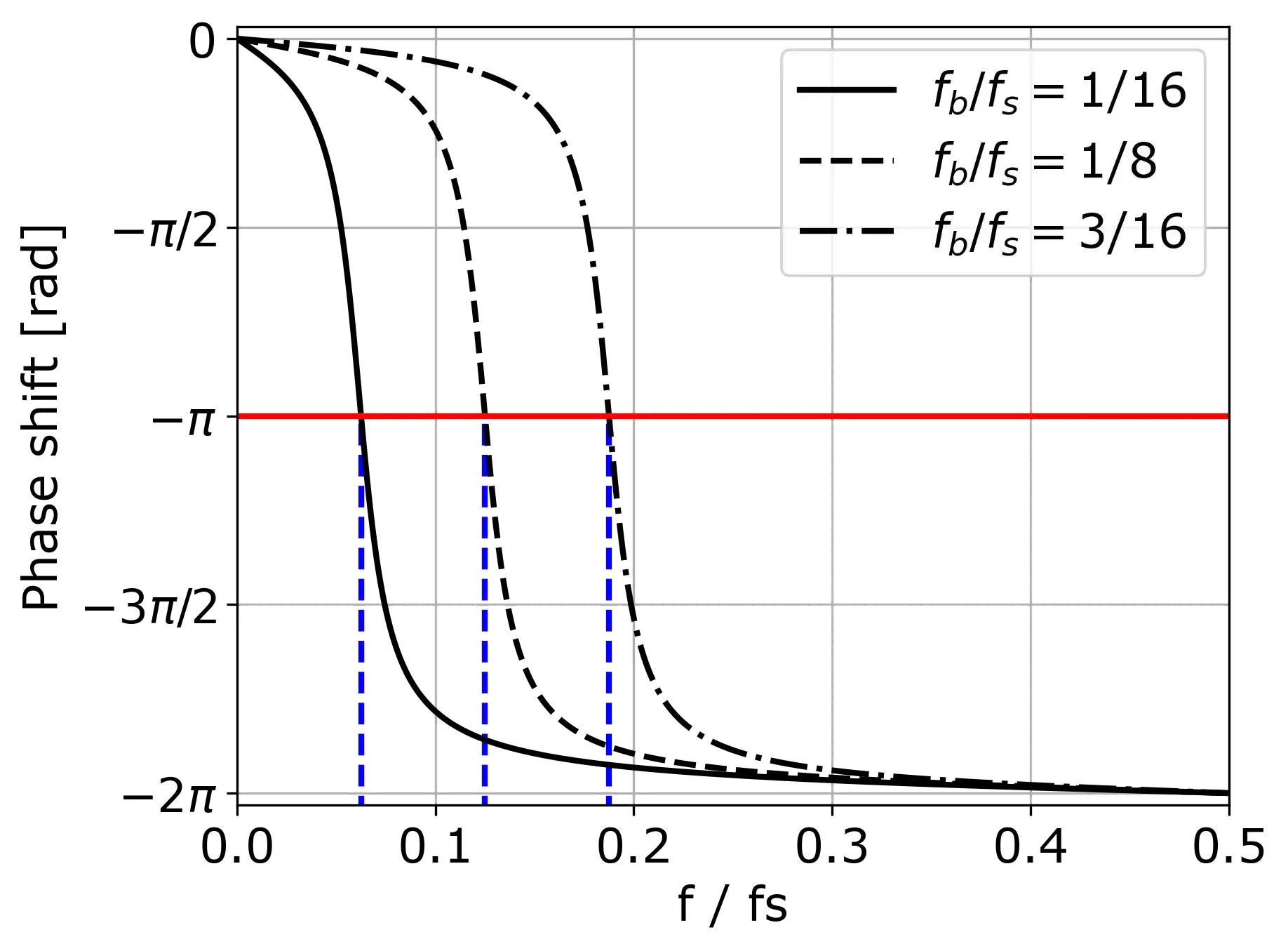Figure 3. Phase response of a second-order allpass filter for different break frequencies frequencies $f_\text{b}$ and bandwidth $BW / f_s = 0.022$.

As you can see, the phase shift is 0 at 0 Hz and gradually changes to $-2\pi$. The steepness of the phase response is determined by the bandwidth $BW$ parameter expressed in Hz.

You can already guess that the bandwidth parameter of the second-order allpass filter translates to the bandwidth parameter of bandpass and bandstop filters. Accordingly, the break frequency corresponds to the center frequency. How?

Thanks to the phase cancellation effect, if we add two tones at the same frequency but with relative phase shift of $\pi$, they will cancel each other. A shift by $\pi$ is equivalent to a multiplication of the tone by -1.

With this knowledge we can now employ the second-order allpass filter for bandpass or bandstop filtering.

## Allpass-Based Bandstop Filter

If we add the output of the second-order allpass filter to its input signal, at the break frequency we will obtain a phase cancellation. Why?

At the break frequency, the phase delay is $-\pi$. Adding two tones at the break frequency with the relative phase shift of $\pi$, we effectively eliminate them from the resulting signal. As the phase shift deviates from $\pi$ further away from the break frequency, the cancellation is less and less effective.

### DSP Diagram

Here is a block diagram of the bandstop filter.Figure 4. DSP diagram of the allpass-based bandstop filter.

$\text{AP}_2(z)$ denotes the second-order allpass filter.

The output of the second-order allpass filter is added to the direct path. We multiply the result by $\frac{1}{2}$ to stay in the [-1, 1] range (input is in [-1, 1] range, allpass’s output is in [-1, 1] range so their sum is in the [-2, 2] range; we want to scale that back down to [-1, 1], otherwise we’ll possibly clip the signal).

### Magnitude Response

Here is a magnitude transfer function of the bandstop filter from Figure 4 with the center frequency at 250 Hz and $Q$ equal to 3.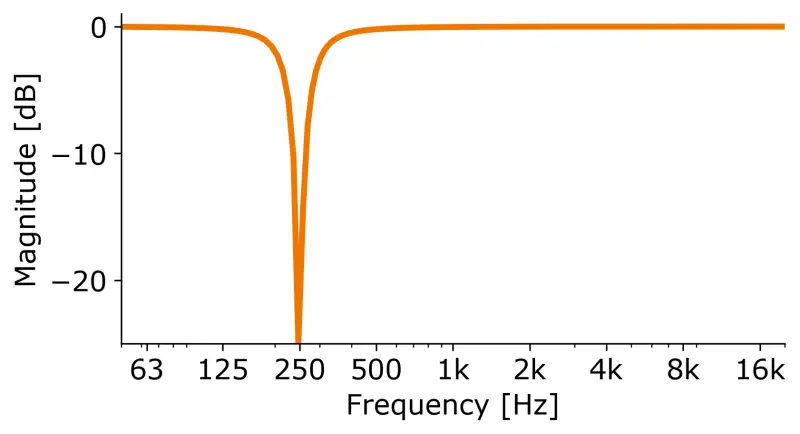Figure 5. Magnitude transfer function of the bandstop filter.

At the center frequency, we get the biggest attenuation which decreases the further away we get from the center frequency. We can see how selective in frequency this filter is.

### Real-Time Control

As this filter requires quite easy computations to control the center frequency and the bandwidth, we can alter its parameters in real time.

As an example, here’s a white noise signal filtered with the bandstop filter, whose center frequency varies from 100 to 16000 Hz over time and Q is equal to 3.

To visualize what’s happening here, take a look at the spectrogram of the audio file.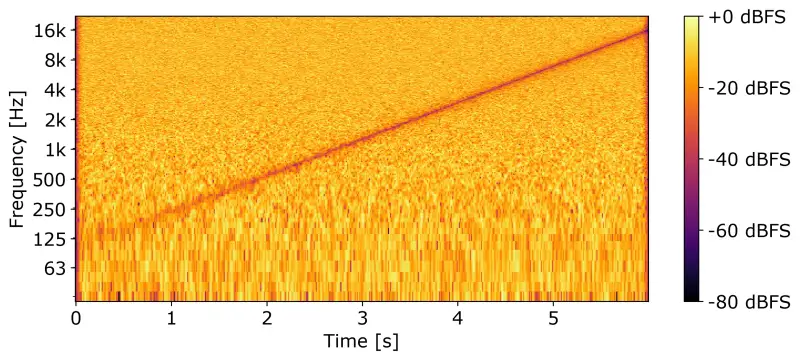Figure 6. Spectrogram of the bandstop filtering example.

On the x-axis we have the time, on the y-axis we have the log-scaled frequency, and color indicates the amplitude level of the frequency at a specific time point in decibels full-scale (dBFS).

As you can see, the dip travels exponentially (mind the log scale!) from low to high frequencies. Thus, we can hear the so-called “filter sweep”.

### Implementation

You will find a sample implementation of the bandstop filter in Python at the end of this article.

## Allpass-Based Bandpass Filter

The allpass-based bandpass filter differs from the bandstop filter only in the sign of the allpass filter output. In case of the bandpass, we invert the output of the allpass in phase so that the phase cancellation occurs at the 0 Hz frequency and the Nyquist frequency. Because the tone at the break frequency gets reversed twice, it is in phase with the input signal. Therefore, the summation results in doubling of the amplitude of the tone corresponding to the break frequency of the allpass.

### DSP Diagram

In Figure 7, there’s a block diagram of the presented bandpass filter.Figure 7. DSP diagram of the allpass-based bandpass filter.

$\text{AP}_2(z)$ denotes the second-order allpass filter.

The multiplication by $\frac{1}{2}$ is just to preserve the [-1, 1] amplitude range of the signal.

### Magnitude Response

In Figure 8, there’s the magnitude response of the bandpass filter with center frequency set to 250 Hz and $Q$ set to 3.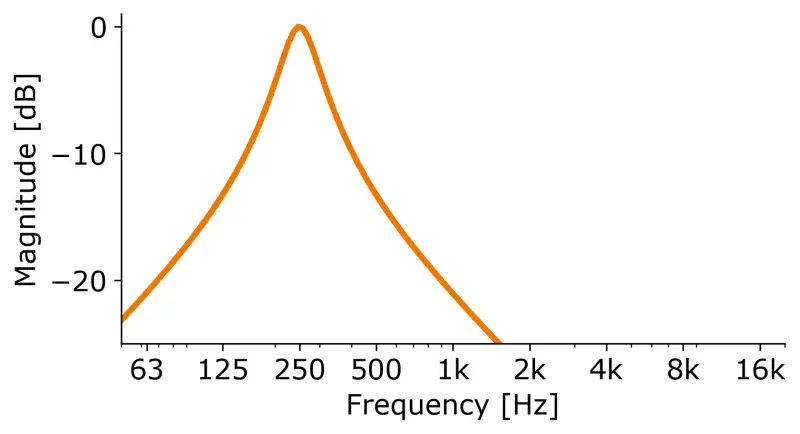Figure 8. Magnitude transfer function of the bandpass filter.

As you can see, it actually passes through only the frequencies in the specified band.

### Real-Time Control

Exactly as the bandstop filter, the bandpass filter can be easily controlled in real time.

Here’s an audio sample with a bandpass-filtered white noise, where the center frequency varies from 100 Hz to 16000 Hz and Q is equal to 3.

You can observe the effect of the bandpass filter on the spectrogram of the above audio file (Figure 9).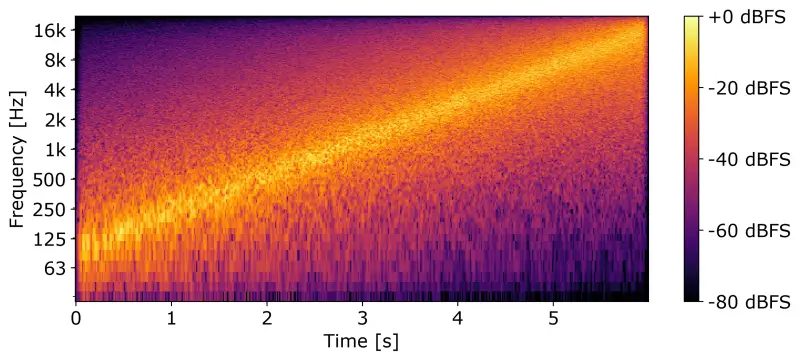Figure 9. Spectrogram of the bandpass filtering example.

Once again, the y-axis is a log-frequency axis, the x-axis is a time axis, and color intensity corresponds to the sound level in decibels full-scale (dBFS).

### Implementation

Here is a sample Python implementation of both filters: the bandpass and the bandstop.

The code generates 5 seconds of white noise and then filters it with time-varying bandstop and bandpass filters respectively. The center frequency in both cases changes exponentially from 100 Hz to 16000 Hz (this code was used to generate the previous examples in this article).

The code is heavily commented so you should have no problems in understanding.

Listing 1. Allpass-based bandstop and bandpass filtering implementation & filter sweep application.

import numpy as np
import scipy.signal as sig
import soundfile as sf

# Use a half-cosine window
window = sig.hann(8192)
# Use just the half of it
# Return the modified signal
return signal

def second_order_allpass_filter(break_frequency, BW, fs):
"""
Returns b, a: numerator and denominator coefficients
of the second-order allpass filter respectively

Refer to scipy.signal.lfilter for the explanation
of b and a arrays.

The coefficients come from the transfer function of
the allpass (Equation 2 in the article).

Parameters
----------
break_frequency : number
break frequency of the allpass in Hz
BW : number
bandwidth of the allpass in Hz
fs : number
sampling rate in hz

Returns
-------
b, a : array_like
numerator and denominator coefficients of
the second-order allpass filter respectively
"""
tan = np.tan(np.pi * BW / fs)
c = (tan - 1) / (tan + 1)
d = - np.cos(2 * np.pi * break_frequency / fs)

b = [-c, d * (1 - c), 1]
a = [1, d * (1 - c), -c]

return b, a

def bandstop_bandpass_filter(input_signal, Q, center_frequency, fs, bandpass=False):
"""Filter the given input signal

Parameters
----------
input_signal : array_like
1-dimensional audio signal
Q : float
the Q-factor of the filter
center_frequency : array_like
the center frequency of the filter in Hz
for each sample of the input
fs : number
sampling rate in Hz
bandpass : bool, optional
perform bandpass filtering if True,
bandstop filtering otherwise, by default False

Returns
-------
array_like
filtered input_signal according to the parameters
"""
# For storing the allpass output
allpass_filtered = np.zeros_like(input_signal)

# Initialize filter's buffers
x1 = 0
x2 = 0
y1 = 0
y2 = 0

# Process the input signal with the allpass
for i in range(input_signal.shape):
# Calculate the bandwidth from Q and center frequency
BW = center_frequency[i] / Q

# Get the allpass coefficients
b, a = second_order_allpass_filter(center_frequency[i], BW, fs)

x = input_signal[i]

# Actual allpass filtering:
# difference equation of the second-order allpass
y = b * x + b * x1 +  b * x2 - a * y1 - a * y2

# Update the filter's buffers
y2 = y1
y1 = y
x2 = x1
x1 = x

# Assign the resulting sample to the output array
allpass_filtered[i] = y

# Should we bandstop- or bandpass-filter?
sign = -1 if bandpass else 1

# Final summation and scaling (to avoid clipping)
output = 0.5 * (input_signal + sign * allpass_filtered)

return output

def main():
"""
Sample application of bandpass and bandstop
filters: filter sweep.
"""
# Parameters
fs = 44100
length_seconds = 6
length_samples = fs * length_seconds
Q = 3

# We have a separate center frequency for each sample
center_frequency = np.geomspace(100, 16000, length_samples)

# The input signal
noise = np.random.default_rng().uniform(-1, 1, (length_samples,))

# Actual filtering
bandstop_filtered_noise = bandstop_bandpass_filter(noise, Q, center_frequency, fs)
bandpass_filtered_noise = bandstop_bandpass_filter(noise, Q, center_frequency, fs,
bandpass=True)

# Make the audio files not too loud
amplitude = 0.5
bandstop_filtered_noise *= amplitude
bandpass_filtered_noise *= amplitude

# Write the output audio file
sf.write('bandstop_filtered_noise.flac', bandstop_filtered_noise, fs)
sf.write('bandpass_filtered_noise.flac', bandpass_filtered_noise, fs)

if __name__=='__main__':
main()



## Applications

Apart from just filtering, bandpass and bandstop filters can be used in a variety of audio effect applications.

### Filter Sweep

Filter sweep is a very strong effect that can add a powerful character to the sound. That’s exactly the effect that you heard in the bandstop-filtering example.

### “Listen” to a Frequency Band (In Audio Plugins)

Audio plugins that use information from a frequency range to control their behavior often have a “listen” functionality that allows you to listen to the frequency band you specified and adjust it.

For example, Tonmann Deesser plugin has the “listen” button to be able to hear the frequency range that is being compressed. With the “listen” functionality we can find the most audible range with the “s” consonant to compress and avoid compressing the desired signal.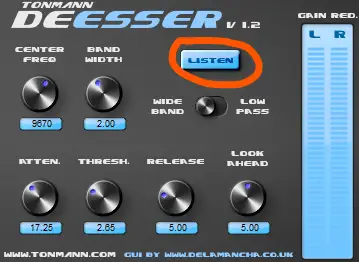Figure 10. Tonmann Deesser plugin has the “listen” functionality.

Alternatively, “listen” can be used to focus on just one part of the spectrum while making edits.

### Phaser

If we modulate the center frequency of the bandstop filter over time, for example, using a low-frequency oscillator (LFO), we can easily obtain the phaser effect.

Better yet, if we have a series of bandstop filters, the effect will be truly awesome! Think Van Halen’s Eruption level of awesome!

The actual application of the phaser effect will be a topic of an another article but you can already experiment with the attached implementation code!

## Summary

In this article, we learned how to implement efficient, real-time-controllable bandpass and bandstop filters using the second-order allpass filter.

Bandpass and bandstop filters are one of the basic effects in the audio programmer’s arsenal. If you want to know which elements make up the audio plugin developer toolbox, check out my free audio plugin developer checklist.